## Short-term Course

Suitable for any grade above III
Duration The duration depends on the list of topics selected by you. The minimum duration is one month.
Choose a subject and/or a list of topics

Choose a subject for a grade and/or a list of topics and we will cover them. The duration of the course depends on the topics included in the course.

You will have a rich experience of learning with the help of programming and visualization. We focus on honing your art of problem solving, and logical and analytical thinking.

All the concepts start from the beginning and we climb the conceptual ladders faster or slower according to the comfort of the students. We assume our students have zero knowledge of any concept and if they already know, we are able to move faster.

## Kids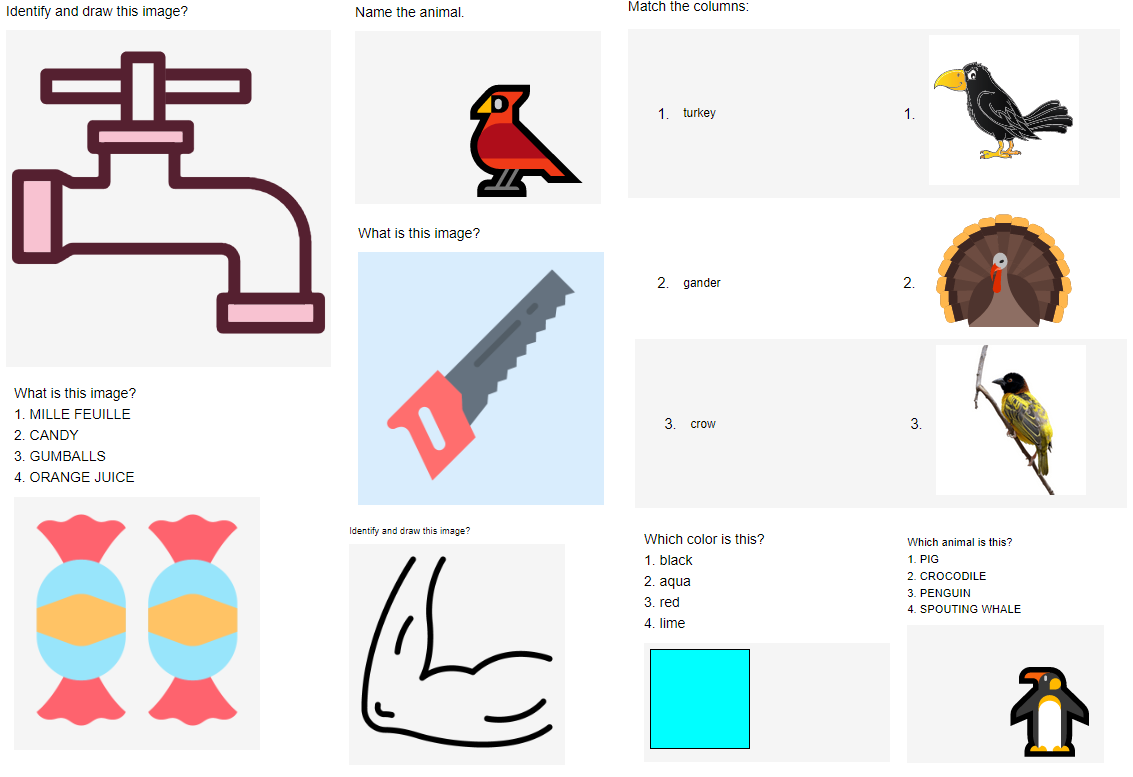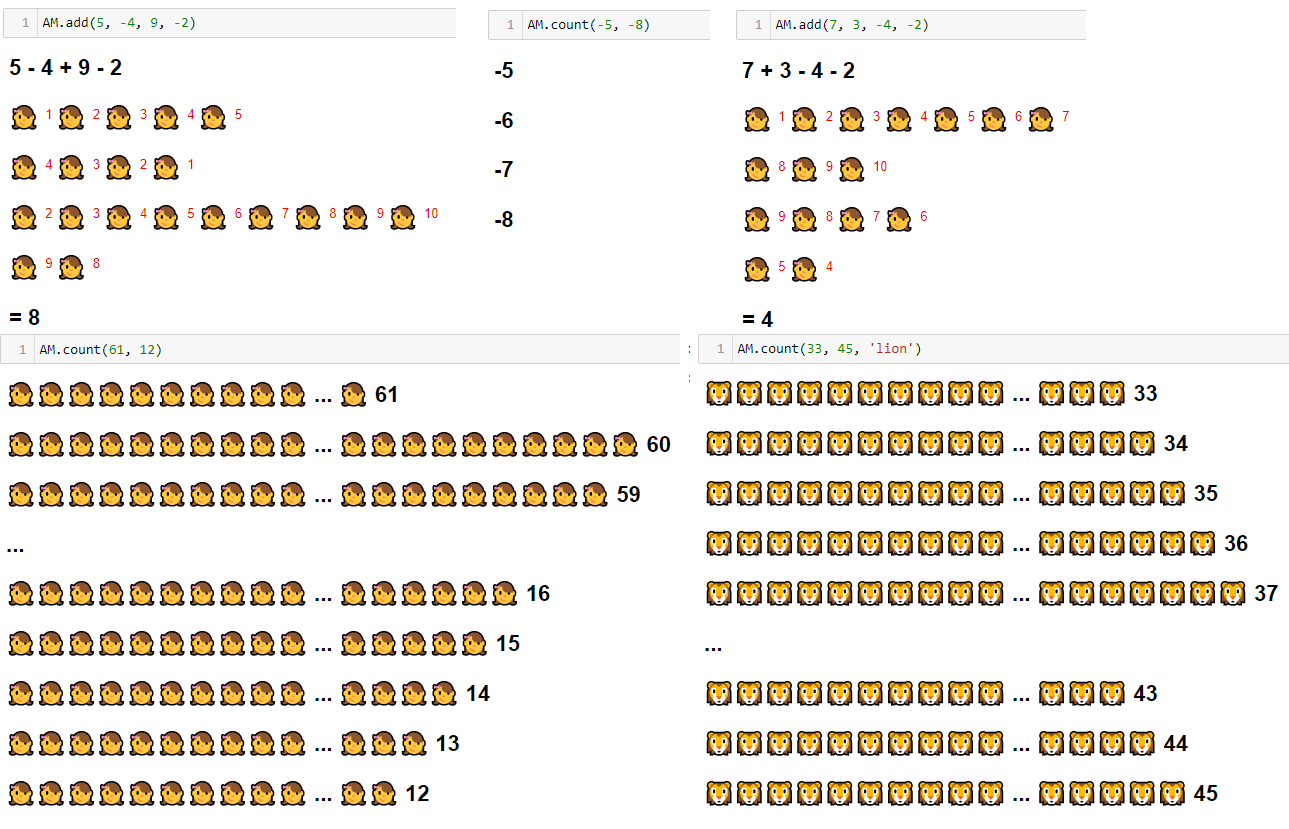Suitable for Grades I and II One parent or a senior person must be available with the student during sessions to help with issues like opening browser, typing, copying and pasting, etc.
Course Duration Regular and paid in advance each month
Course Content
1. Counting, steps and patterns
3. Various types of lines and shapes
4. Simple programs related to shapes and patterns
5. Open and closed spaces
6. Find basic geometric shapes and forms in their world—plants, animals, figures, etc.
8. Division, fraction
9. Identify and draw images
10. Analyze the similarities and differences
11. Identify details
12. Recognize primary and secondary colors; colors that represent things and ideas.
13. Clocks, calendars and currencies
14. Units and measurement
15. Learn and use new vocabulary
16. Identifying the meaning of words in a variety of texts
17. Characters and plots in a story
A peep into what our students do in these grades:

The section below lists out some of activities related to students of this grade.

### kindergarten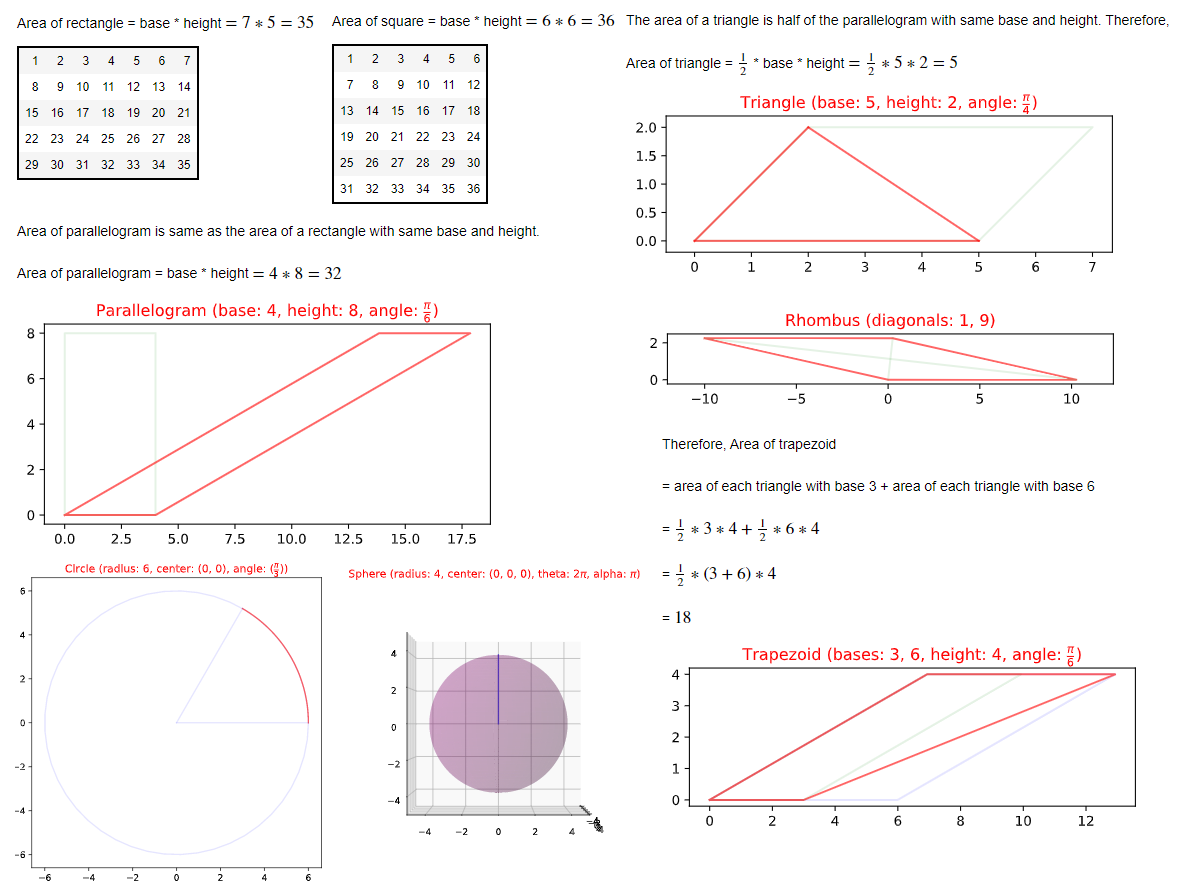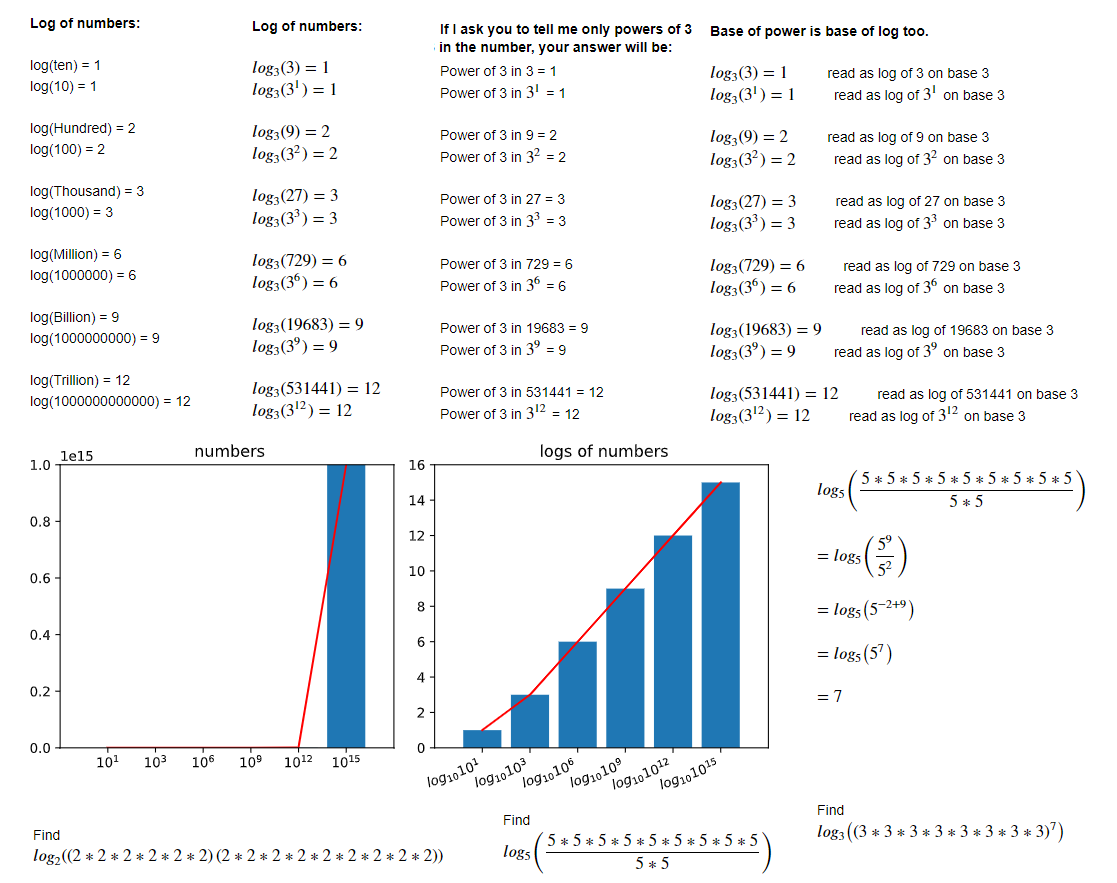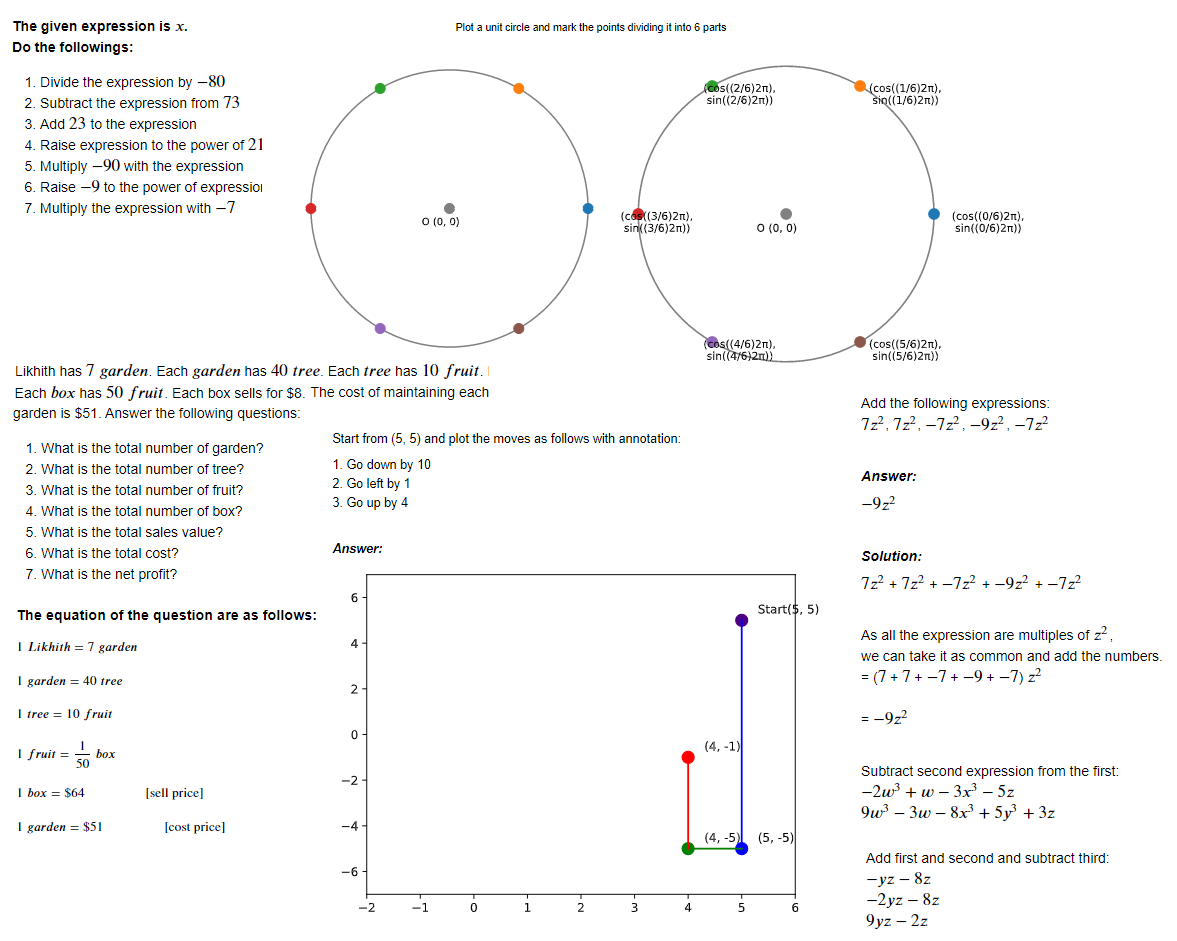Suitable for Grade III, IV, V

This is the lowest grade we serve. The students must have knowlegde of internet, should be able to open links, click icons, copy and paste text, understand left and right clicks and use bookmark to access our software platform in browser.

Course Duration Regular and paid in advance each month
Course Content

Programming and visualization are essential part of our course content. With plotting charts and graphs of various types, students learn the concepts listed below which are inclusive, not exhaustive. Students are also encouraged to bring their own topics, including the ones they might be studying in school during that time.

All the concepts start from the beginning and we climb the conceptual ladders faster or slower according to the comfort of the students. We assume our students have zero knowledge of any concept and if they already know, we are able to move faster.

1. Plotting and visualization of points, circles without sin, cos
2. Animation of basic shapes like points and stars
3. Applied plotting of various types of charts like bar, pie, line etc.
4. Plotting unit circle, concept of angle, radius, pi, degree, cos as left/right and sin as up/down
5. Place value digits in integers, fractions and decimals
6. Addition, subtraction, multiplication and division of integers, fraction and decimals
7. Estimation of numbers
8. Estimated addition, subtraction, multiplication and division of numbers
9. Scientific expression of numbers
10. Arithmetic operations with multiple operators and groups
11. Simple divisibility tests
12. Factors, prime numbers, coprimes, LCM and HCF
13. Concept of power (exponent)
14. Inequality of numbers
15. Law of proportion and verbal problems
16. Geometrical shapes, their classifications and properties
17. Area, perimeter and volumes of basic geometrical shapes
18. Units of measurements and their conversions
19. Concept of difference between two sets of numbers
20. Series and tabular data, mean, median and frequency
21. Algebric expressions
22. Solving equations and writing them in concise (matrix) form
A peep into what our students do in these grades: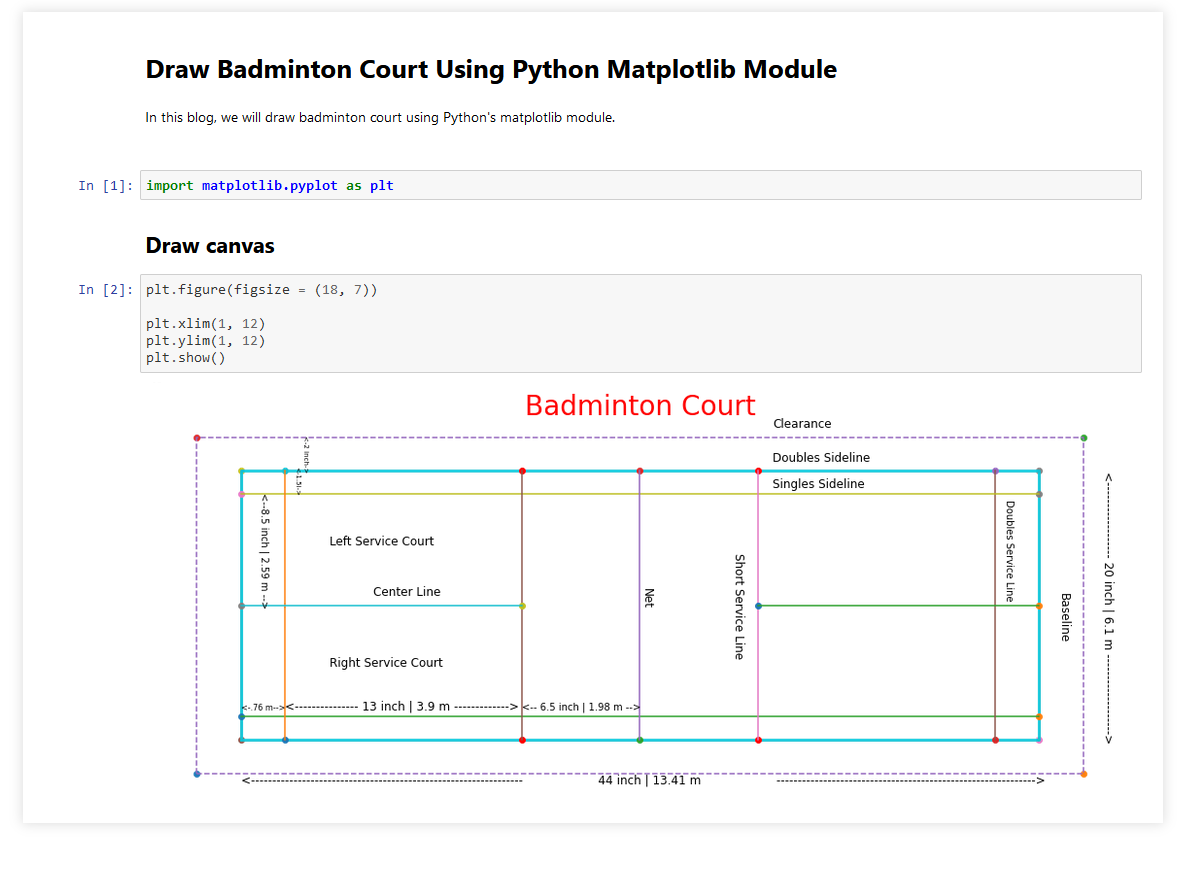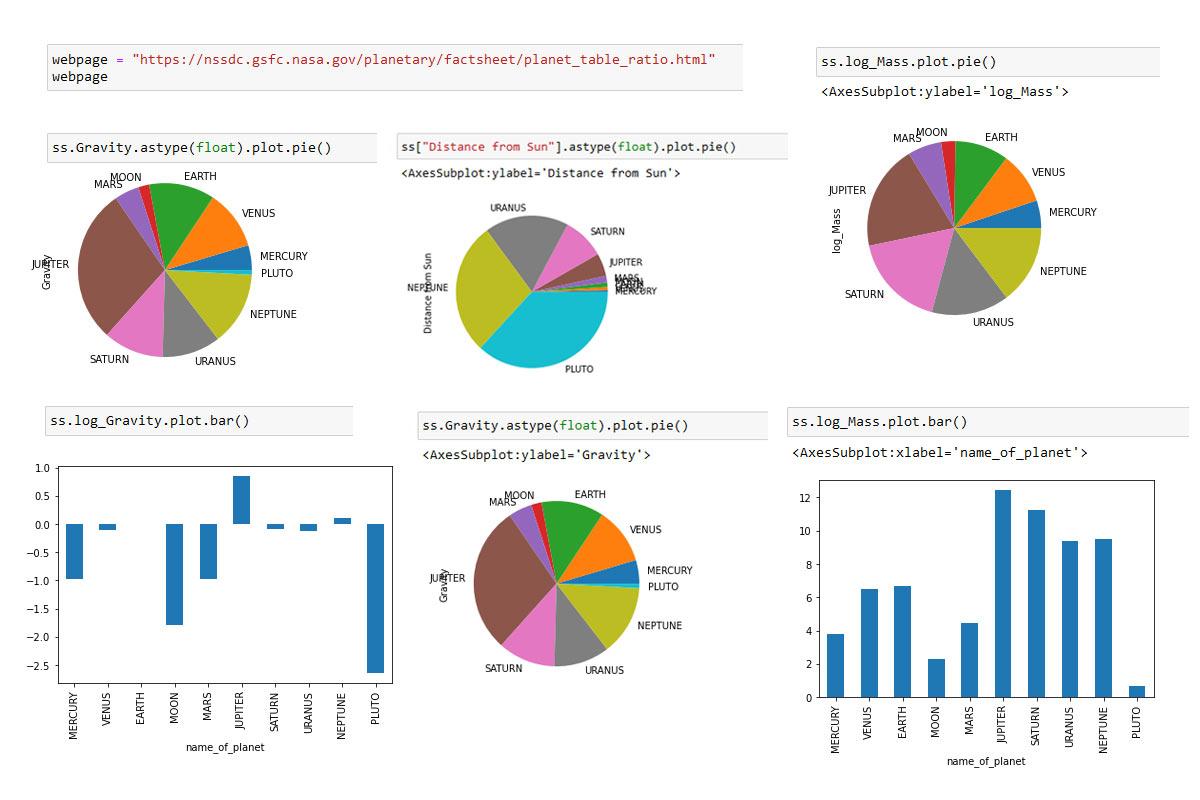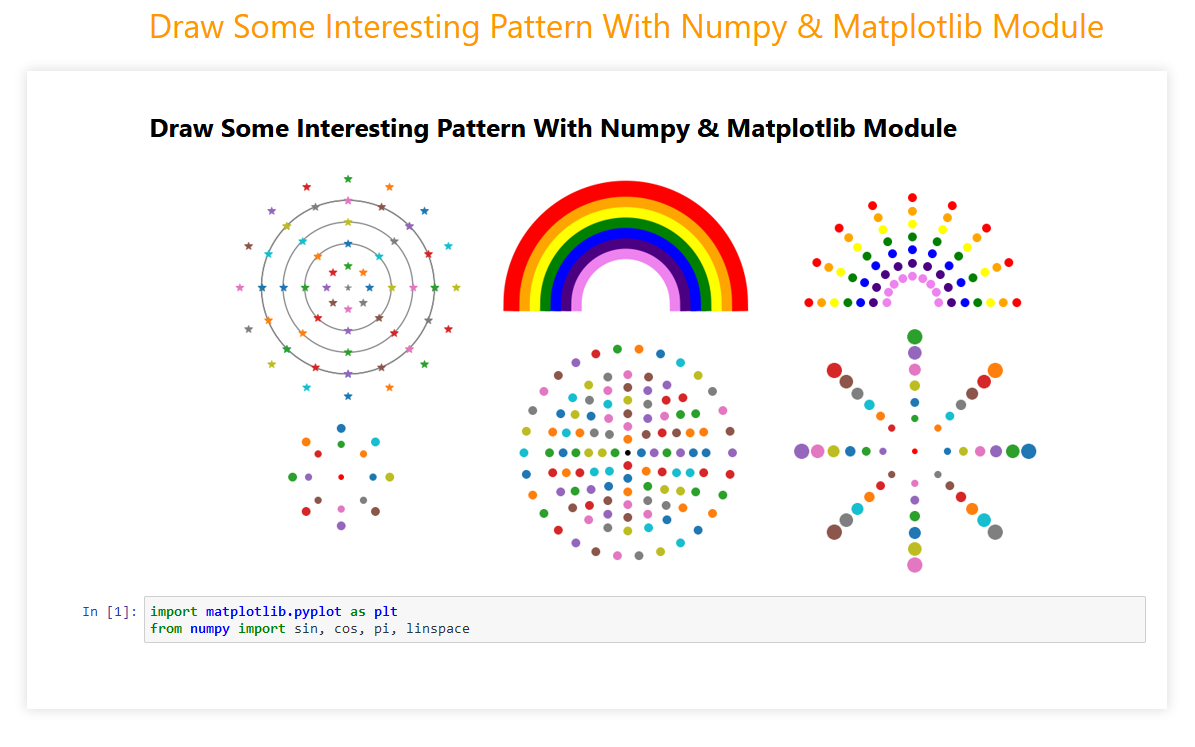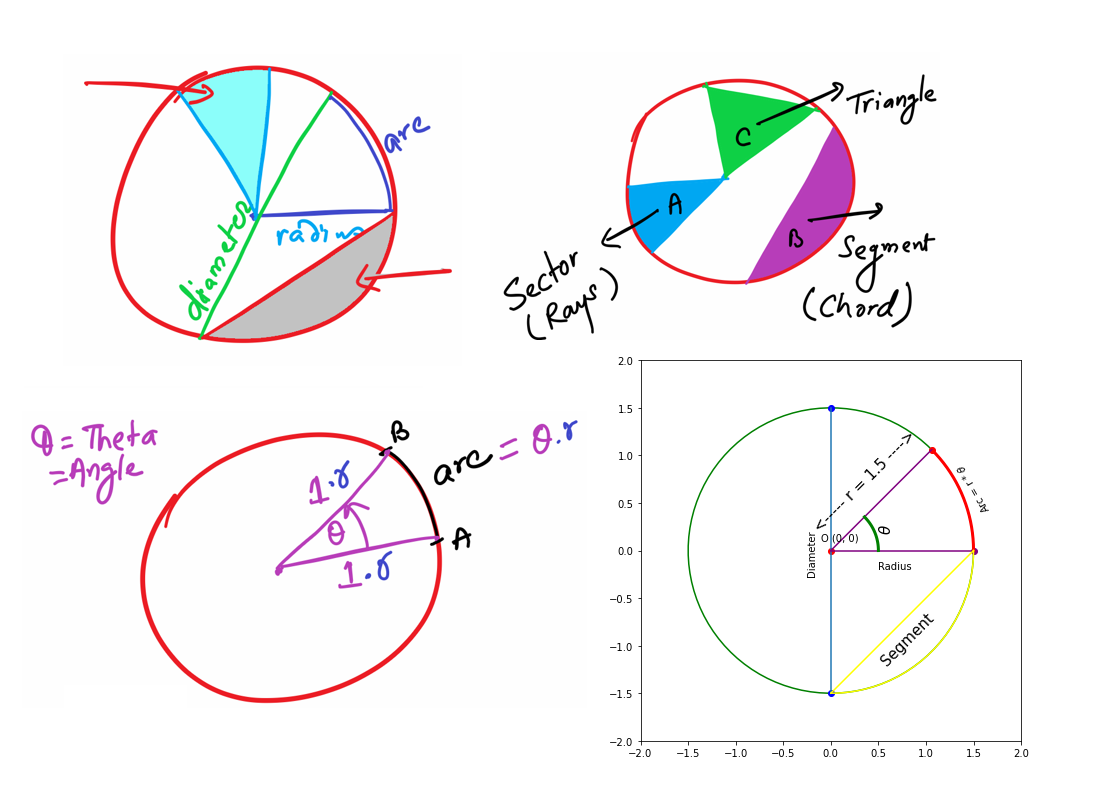Suitable for grade VI, VII and VIII
Course Duration Regular and paid in advance each month
Course Content

Programming and visualization are essential part of our course content. With plotting charts and graphs of various types, students learn the concepts listed below which are inclusive, not exhaustive. Students are also encouraged to bring their own topics, including the ones they might be studying in school during that time.

All the concepts start from the beginning and we climb the conceptual ladders faster or slower according to the comfort of the students. We assume our students have zero knowledge of any concept and if they already know, we are able to move faster.

1. Lines, line segments, circles, rays
2. Curves and polygons
3. Angles, Types of angles
4. Measuring line segments and angles
5. Parallel and perpendicular lines
6. Classification of triangles
8. Fractions on the number line
9. Improper, mixed fractions, equivalent fractions and comparing fractions
10. Addition or subtracting of numbers with decimals
11. Area, perimeter and volumes of basic geometrical shapes
12. Expressions with variables and solution of an equation
13. Adding, subtracting, multiply & divide negative numbers
14. Adding, subtracting, multiply & divide negative fractions
15. Converting fractions to decimals
16. Constant of proportionality
17. The distributive property & equivalent expressions
18. Linear expressions
19. Area and circumference of circles
20. Vertical, complementary, and supplementary angles
21. Slicing geometric shapes
22. Inequality of numbers
23. Probability
24. Square roots & cube roots
25. Exponents with negative bases, exponent properties, negative exponents
26. Arithmetic with numbers in scientific notation
27. Intercepts, slope
28. Angles between intersecting lines, triangle angles
29. Pythagorean theorem
30. Translations
31. Rotations
32. Reflections
33. Dilations
34. Congruence and similarity
35. Plotting and visualization of scatter plots, estimating lines of best fit
A peep into what our students do in these grades:

## High School

Suitable for Grade IX, X, XI, XII
Course Duration Regular and paid in advance each month
Course Content

Programming and visualization are essential part of our course content. With plotting charts and graphs of various types, students learn the concepts listed below which are inclusive, not exhaustive. Students are also encouraged to bring their own topics, including the ones they might be studying in school during that time.

All the concepts start from the beginning and we climb the conceptual ladders faster or slower according to the comfort of the students. We assume our students have zero knowledge of any concept and if they already know, we are able to move faster.

1. Introduction to Euclidean geometry
2. Introduction to rigid transformations
3. Transformation properties and proofs
4. Translations
5. Rotations
6. Reflections
7. Dilations
8. Transformations & congruence
9. Triangle congruence from transformations
10. Congruent triangles
11. Theorems concerning triangle properties
13. Proofs of general theorems
14. Constructing lines & angles
15. Definitions of similarity
16. Introduction to triangle similarity
17. Angle bisector theorem
18. Pythagorean theorem and proofs
19. Sine & cosine of complementary angles
20. Ratios in right triangles
21. Distance and midpoints
22. Dividing line segments
23. Equations of parallel & perpendicular lines
24. Graphs of circles intro
25. Standard equation and Expanded equation of a circle
26. Focus and directrix of a parabola
27. Circle basics
28. Arc measure, Arc length (from degrees), Arc length (from radians)
29. Sectors, Inscribed angles
30. Properties of tangents
31. Constructing circumcircles & incircles
32. Cavalieri's principle and dissection methods
33. Volume and surface area
34. Density
35. Frequency tables and dot plots
36. Histograms, box, whisker and scatter plots
37. Mean and median in data displays and Interquartile range
38. Standard deviation
39. Comparing distributions
40. Percentiles and z-scores
41. Normal distributions and the empirical rule
42. Normal distribution calculations
43. Fitting trend lines to scatterplots
44. Introduction to planning a study
45. Introduction to experimental design
46. Probability, Permutations and Combinations
47. Venn diagrams and the addition rule
48. Probability using combinatorics
49. Probability distributions & expected value
A peep into what our students do in these grades:

## College

Suitable for Freshers and experienced professionals who want to build/change their career in Machine Learning and Data Science, even the beginners who have little or no experience of Python and Machine Learning.
Course Duration 120 working hours or 60 working days or 90 calendar days
Course Content
• check Starting from basic of Python, the course covers advanced packages such as Numpy, Scipy, Pandas etc.
• check It covers data visualization tools like Matplotlib, Seaborn, ggplot and geoplotlib. It covers concepts of machine learning from basic to advanced and uses the libraries like Sklearn, Keras, TensorFlow, NLTK, RASA etc. It also covers use cases from Kaggle competitions to give real-life problem-solving experience.
• check To help people with less inclination to programming, we teach GUI based tools like Knime, Weka and Orange.
• check This course goes on to cover the live deployment in cloud and creating end-to-end pipeline of a real-life machine learning project. We use popular editing tools like Eclipse and Jupyter Notebook.
A peep into what our students do in these grades:

## Mathematics

If you like problem solving and logical thinking, this is for you.

The course focuses on mathematics, starting from primary school mathematics to high school mathematics for students who are planning to appear in tests like A-level, STEP, Math Olympiad etc.

Our software ensures to quickly identifies your current level of knowledge and conceptual base and takes it forward from there. So, a student can join this course irrespective of the grade one belongs to.

Course Duration Long term. Paid monthly/quarterly/semi-annually/annualy in advance.
Course Content

This course covers all mathematical concepts up to senior high school level. The AI models ensure, with help of networked concept maps, that the students learn related concepts quickly, in the easiest and shortest possible time.

The content takes its own path depending on appetite and interest of the students.

A peep into what our students do in these grades:

## Physics

Suitable for students who like Physics and Math

The students must have knowlegde of internet, should be able to open links, click icons, copy and paste text, understand left and right clicks and use bookmark to access our software platform in browser.

Course Duration Regular and paid in advance each month
Course Content

Programming and visualization are essential part of our course content. With plotting charts and graphs of various types, students learn the concepts listed below which is inclusive, not exhaustive. Students are also encouraged to bring their own topics, including the ones they might be studying in school during that time.

All the concepts start from the beginning and we climb the conceptual ladders faster or slower according to the comfort of students. We assume our students to have zero knowledge in any concept and if they already know, we are able to move faster.

1. Introduction to physics
2. Acceleration, Displacement, velocity, and time
3. Kinematic formulas and projectile motion
4. One-dimensional motion and Two-dimensional motion
5. Forces and Newton's laws of motion
6. Normal force, contact force, balanced and unbalanced forces
7. Inclined planes and friction
8. Tension
9. Centripetal force and gravitation
10. Circular motion and centripetal acceleration
11. Newton's law of gravitation
12. Work and energy
13. Springs and Hooke's law
14. Impacts and linear momentum
15. Momentum and Impulse
16. Elastic and inelastic collisions
17. Torque, moments, and angular momentum
18. Rotational kinematics
19. Oscillations and mechanical waves
20. Simple harmonic motion
21. Introduction to mechanical waves
22. Sound: Oscillations and mechanical waves
23. The Doppler effect
24. Wave interference
25. Fluid Dynamics
26. Density and Pressure
27. Buoyant Force and Archimedes' Principle
28. Laws of thermodynamics
29. Temperature, kinetic theory, and the ideal gas law
30. Specific heat and heat transfer
31. Electric charge, field, and potential
32. Charge and electric force (Coulomb's law)
33. Electric potential energy, electric potential, and voltage
34. Ohm's law and circuits with resistors
35. Magnetic forces, magnetic fields, and Faraday's law
36. Introduction to Electromagnetic waves and interference
37. Geometric optics
38. Reflection and refraction
39. Michelson and Morley's luminiferous ether experiment
40. Quantum Physics
41. Photons, atoms and electrons
42. Quantum numbers and orbitals
43. Cosmology and astronomy
44. Stars, black holes and galaxies
45. Life on earth and in the universe
A peep into what our students do in these grades:

## Chemistry

Suitable for students who like Chemistry

The students must have knowlegde of internet, should be able to open links, click icons, copy and paste text, understand left and right clicks and use bookmark to access our software platform in browser.

Course Duration Regular and paid in advance each month
Course Content

Programming and visualization are essential part of our course content. With plotting charts and graphs of various types, students learn the concepts listed below which is inclusive, not exhaustive. Students are also encouraged to bring their own topics, including the ones they might be studying in school during that time.

All the concepts start from the beginning and we climb the conceptual ladders faster or slower according to the comfort of students. We assume our students to have zero knowledge in any concept and if they already know, we are able to move faster.

1. Atoms, compounds, and ions
2. Names and formulas of ionic compounds
3. Moles and molar mass
4. Isotopes
5. Mixtures
6. Mass spectrometry
7. molecular composition
8. Balancing chemical equations and types of chemical reactions
9. Stoichiometry
10. Limiting reagent stoichiometry
11. Molecular composition
12. Net ionic equations
13. Representations of reactions
14. Physical and chemical changes
15. History of atomic structure
16. Bohr's model of the hydrogen atom
17. Quantum numbers and orbitals
18. Electron configurations
19. Introduction to the periodic table
20. Periodic table trends
21. Types of chemical bonds
22. Bond energy, Solids
23. Dot structures and molecular geometry
24. Hybridization and hybrid orbitals
25. Ideal gas equation
26. Kinetic molecular theory
27. Non-ideal gas behavior
28. States of matter and intermolecular forces
29. Mixtures and solutions
30. Separating mixtures and solutions
31. Equilibrium constant
32. Acids, bases, and pH
33. Acid-base equilibria
34. Buffers, titrations, and solubility equilibria
35. Thermodynamics
36. Internal energy, Enthalpy, Entropy, Gibbs free energy
37. Oxidation-reduction reactions
38. Galvanic cells and standard cell potentials
39. Electrochemistry, thermodynamics, and equilibrium
40. Cell potentials under nonstandard conditions
41. Electrolytic cells and electrolysis
42. Reaction rates and rate laws
43. Relationship between reaction concentrations and time
44. Arrhenius equation and reaction mechanisms
45. Spectroscopy
47. Alkanes, cycloalkanes, and functional groups
48. Naming alkanes, cycloalkanes, and bicyclic compounds
49. Conformations of alkanes and cycloalkanes
50. Functional groups
A peep into what our students do in these grades:

## Programming and Visualization for Math & Science

Suitable for all who want to use programming to enrich their learning experience

This is the lowest grade we serve. The students must have knowlegde of internet, should be able to open links, click icons, copy and paste text, understand left and right clicks and use bookmark to access our software platform in browser.

Course Duration Regular and paid in advance each month
Course Content

Programming and visualization are essential part of our course content. With plotting charts and graphs of various types, students learn the concepts listed below which is inclusive, not exhaustive. Students are also encouraged to bring their own topics, including the ones they might be studying in school during that time.

All the concepts start from the beginning and we climb the conceptual ladders faster or slower according to the comfort of students. We assume our students to have zero knowledge in any concept and if they already know, we are able to move faster.

1. Plotting and visualization of points, circles without sin, cos
2. Animation of basic shapes like points and stars
3. Applied plotting of various types of charts like bar, pie, line etc.
4. Plotting unit circle, concept of angle, radius, pi, degree, cos as left/right and sin as up/down
5. Place value digits in integers, fractions and decimals
6. Addition, subtraction, multiplication and division of integers, fractions and decimals
7. Estimation of numbers
8. Estimated addition, subtraction, multiplication and division of numbers
9. Scientific expression of numbers
10. Arithmetic operations with multiple operators and groups
11. Simple divisibility tests
12. Factors, prime numbers, coprimes, LCM and HCF
13. Concept of power (exponent)
14. Inequality of numbers
15. Law of proportion and verbal problems
16. Geometrical shapes their classifications and properties
17. Area, perimeter and volumes of basic geometrical shapes
18. Units of measurements and their conversions
19. Concept of difference between two sets of numbers
20. Series and tabular data, mean, median and frequency
21. Algebric expressions
22. Solving equations and writing them in concise (matrix) form
A peep into what our students do in these grades: